#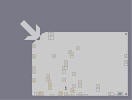Hover over the thumbnail for a full-size version.

Author wedgie123 author:wedgie123 dda rated v1.3c 2004-12-24 4 by 32 people. \$Powered by bounce blocks? Extended#wedgie123#none#11111111111111111111111111111111111111111111111111111111111111111111111111111111111111111111111152111111111111111111111402151111111111111111111402011111111111111111111400500000000000001111140030000000000000011111115000000000000000111111100000000000000001111111000000000000000011111110000000000000000111111100000000000000001111111000000000000000011111110000000000000000111111100000000000000001111111000000000000000011111110000000000000000111111100000000000000001111111000000000000000011111110000000000000000111111100000000000000001111111000000000000000011111110000000000000000111111100000000000000001111111000000000000000011111110000000000000000111111100000000000000001111111000000000000000011111110000000000000000|5^396,528!1^396,564!1^396,564!1^396,564!1^396,564!1^396,564!0^396,564!0^396,564!0^396,564!0^396,564!0^396,564!0^396,564!0^396,564!1^384,480!0^384,480!0^384,480!0^384,480!0^384,480!0^384,480!0^384,480!0^384,480!0^384,480!1^444,516!1^444,516!1^444,516!1^444,516!1^444,516!0^444,516!0^444,516!1^444,444!0^444,444!0^444,444!0^444,444!0^444,444!0^444,444!1^660,564!1^660,564!1^660,564!1^660,564!1^660,564!1^660,564!1^660,564!1^660,564!1^660,564!1^660,564!1^660,564!1^660,564!1^660,564!1^660,564!0^660,564!0^660,564!0^660,564!0^660,564!0^660,564!0^660,564!0^660,564!0^660,564!0^660,564!0^660,564!0^660,564!0^660,564!0^660,564!0^660,564!0^660,564!1^672,564!1^672,564!1^516,576!1^516,576!0^516,576!0^516,576!0^516,576!0^516,576!0^516,576!0^516,576!0^516,576!0^516,576!0^516,576!0^516,576!0^516,576!1^492,576!0^492,576!0^492,576!0^492,576!0^492,576!0^492,576!0^492,576!1^468,576!1^468,576!1^468,576!1^468,576!1^468,576!1^468,576!1^468,576!1^468,576!1^468,576!1^468,576!1^468,576!1^468,576!1^480,564!1^480,564!1^480,564!1^480,564!1^480,564!1^480,564!1^480,564!1^480,564!1^480,564!0^480,564!0^480,564!0^480,564!0^480,564!0^480,564!0^480,564!0^480,564!1^480,516!1^480,516!1^480,516!1^480,516!1^480,516!1^480,516!1^480,516!0^480,516!0^480,516!0^480,516!0^480,516!0^480,516!0^480,516!0^480,516!0^480,516!0^444,516!0^444,516!0^444,516!0^444,516!1^480,492!1^480,492!1^480,492!0^480,492!0^480,492!0^480,492!0^480,492!1^468,288!1^468,288!0^468,288!0^468,288!0^468,288!0^468,288!0^468,288!0^468,288!1^756,336!0^756,336!0^756,336!0^756,336!0^756,336!0^756,336!0^756,336!0^756,336!1^756,372!1^756,372!1^756,384!1^756,384!1^756,372!0^756,372!0^756,372!0^756,372!0^756,372!0^756,372!0^756,372!0^756,372!0^756,336!0^756,336!0^756,336!0^756,336!0^756,336!0^756,336!1^768,336!3^756,204!3^756,564!3^264,192!3^192,576!1^588,528!1^588,540!1^588,540!1^588,516!1^588,516!1^588,516!1^588,516!1^588,516!1^588,516!1^588,516!1^588,516!1^588,516!0^588,516!0^588,516!0^588,516!0^588,516!0^588,516!0^588,516!0^588,516!0^588,516!0^588,516!0^588,516!1^432,576!1^432,576!1^432,576!1^432,576!1^432,576!0^432,576!0^432,576!0^432,576!0^432,576!0^432,576!0^432,576!1^420,576!1^420,576!0^420,576!0^420,576!0^420,576!0^420,576!0^420,576!0^420,576!0^420,576!0^420,576!0^420,576!1^432,540!1^432,540!0^432,540!0^432,540!0^432,540!0^432,540!0^432,540!0^432,540!0^432,540!0^444,540!0^444,540!0^444,552!0^444,552!0^432,552!0^432,552!0^432,540!0^432,540!0^432,540!1^360,564!1^360,564!1^360,564!1^360,564!0^360,564!0^360,564!0^360,564!0^360,564!0^360,564!0^360,564!0^360,564!0^360,564!0^360,552!0^360,552!0^360,552!0^360,552!0^360,552!0^372,552!0^372,552!0^372,552!0^372,552!0^372,552!0^372,564!0^372,564!0^372,564!0^372,564!0^372,564!0^372,564!0^372,564!1^312,576!1^312,576!1^312,576!0^312,576!0^312,576!0^312,576!0^312,576!0^312,576!0^312,576!0^312,576!0^312,576!1^300,528!0^300,528!0^300,528!0^300,528!0^300,528!0^300,528!0^300,528!0^300,528!0^300,528!0^300,528!0^300,528!0^300,528!1^300,504!1^300,504!0^300,504!0^300,504!0^300,504!0^300,504!0^300,504!0^300,504!0^300,504!0^300,504!0^300,504!0^300,504!0^300,504!0^300,504!0^300,516!0^300,516!0^300,516!0^300,516!0^300,504!0^300,504!1^288,480!1^288,480!0^288,480!0^288,480!0^288,480!0^288,480!0^288,480!0^288,480!0^288,480!0^288,480!0^288,480!0^288,480!0^300,480!0^300,480!0^300,480!0^300,480!1^288,456!1^288,456!1^288,456!1^288,456!0^288,456!0^288,456!0^288,456!0^288,456!0^288,456!0^288,456!0^288,456!0^288,456!0^288,456!0^288,456!1^228,576!1^228,576!1^228,576!1^228,576!1^228,576!0^228,576!0^228,576!0^228,576!0^228,576!0^228,576!0^228,576!0^228,576!0^228,576!0^228,576!0^228,576!1^216,564!1^216,564!1^216,564!1^216,564!0^228,564!0^228,564!0^228,564!0^228,564!1^252,564!1^252,564!1^252,564!1^252,564!0^252,564!0^252,564!0^252,564!0^252,564!0^252,564!0^252,564!0^252,564!0^252,552!0^252,552!0^240,552!0^240,552!0^240,564!0^240,564!1^228,564!1^228,564!1^228,564!1^228,564!1^228,564!1^228,564!1^228,564!0^228,564!0^228,564!0^228,564!0^228,564!0^228,564!0^228,564!0^228,564!0^228,564!0^228,564!0^228,564!0^228,564!0^228,564!0^228,564!0^228,564!0^228,564!0^228,564!0^228,564!0^228,564!0^228,564!0^228,564!0^228,564!1^204,480!1^204,480!1^204,480!1^204,480!0^204,492!0^204,480!0^204,480!0^204,480!0^204,480!0^204,480!0^204,480!0^204,480!0^204,480!1^204,432!1^204,432!0^204,432!0^204,432!0^204,432!0^204,432!0^204,432!0^204,432!0^204,432!0^204,432!0^204,432!0^204,432!1^204,408!1^204,408!1^204,408!1^204,408!0^204,408!0^204,408!0^204,408!0^204,408!0^204,408!0^204,408!0^204,408!0^204,408!0^204,408!0^204,408!1^192,336!1^192,336!1^204,336!1^204,336!1^204,336!1^204,336!1^204,336!1^204,336!0^204,336!0^204,336!0^204,336!0^204,336!0^204,336!0^204,336!0^204,336!0^204,336!0^204,336!0^204,348!0^204,348!0^204,348!0^204,348!1^204,276!1^204,276!0^204,276!0^204,276!0^204,276!0^204,276!0^204,276!0^204,276!0^204,276!0^204,276!0^204,276!0^204,276!1^324,336!0^324,336!0^324,336!0^324,336!0^324,336!0^324,336!0^324,336!1^396,372!1^396,372!0^396,372!0^396,372!1^384,408!1^384,408!1^384,420!1^384,420!1^384,420!1^384,420!1^384,408!0^384,408!0^384,408!0^384,408!0^384,408!0^384,408!0^384,408!1^396,396!1^396,396!1^396,396!1^396,408!1^396,408!1^396,408!0^396,396!0^396,396!0^396,396!0^396,396!0^396,396!0^396,396!0^396,396!1^384,432!1^384,432!1^384,432!1^384,432!1^384,432!1^384,432!1^384,432!1^384,432!1^384,432!1^384,432!1^300,204!1^300,204!1^300,204!1^300,204!1^312,204!1^312,204!1^312,204!0^312,204!0^312,204!0^312,204!0^312,204!0^312,204!0^312,204!0^312,204!0^312,204!1^300,228!1^300,228!1^300,228!1^300,228!1^300,228!1^300,228!1^300,228!1^300,228!1^300,228!1^300,228!1^300,228!1^312,228!1^312,228!1^312,228!1^312,228!1^312,228!1^312,228!1^312,228!1^312,228!1^312,228!1^312,228!1^420,312!1^420,312!1^420,312!1^420,312!0^420,312!0^420,312!0^420,312!0^420,312!0^420,312!0^420,312!1^432,336!1^432,336!1^432,336!1^432,336!1^432,336!1^432,336!1^432,324!1^432,324!1^432,324!1^432,324!0^432,324!0^432,324!0^432,324!0^432,324!1^696,576!1^696,576!9^720,564,0,1,31,23,0,-1,0!9^720,564,1,1,31,9,0,0,-1!9^720,564,1,1,14,10,0,0,0!9^720,564,0,1,11,23,0,0,0!11^720,564,720,564# I just made this a bit longer, hope you still like it.

## Other maps by this author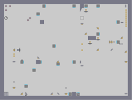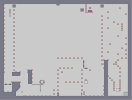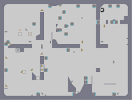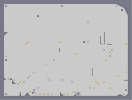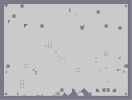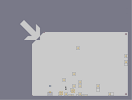My first DDA Elevator - part 2 that time again Door DDA - version 2 i love goooooooold Powered by bounce blocks? (fixed)

Pages: (0)

### You know what?

I've always wanted to make one of these.
This got a bit boring after a while, bt hey, 3.5

### i like it

It is a unique idea, fun to watch the first time.

It lacks more enemies. Awesome map, 4.5/5!

### hmm

isnt the point of a dda to be collecting everything? u only got like half the gold lol

### ...

how do u do that la?

### lol sorry

the first version was more of a trial, but when i saw how much people liked it i made this extended version, but it wouldnt let me update the map, so i posted it again.

### Hmm...

I'm not a fan of voting for two versions of the same map, I would expect no less of any of you with the maps that I have duplicates of. So, I'ma abstain my vote from this version.

### map is awesome

still fascinating!

### oops

i mean here http://numa.notdot.net/map/4582

### ..

this is the extended version, this is the original http://numa.notdot.net/map/4582. please vote this too if you've allready voted on the other one lol ;)